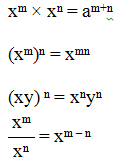# Tricks to Solve Surds and Indices Questions

#### Ques 1:

Solve: 360.14 x  12960.18
Solution:
Given that ab x ac = a (b+c)
According to the question and as per the formula given above:
360.14 x 12960.18 (Make factors of 1296 = 36x36 i.e. equalizing the bases of the two figures)
360.14 x (362)0.18

360.14 x 360.36
36(0.14 + 0.36)
360.5
36(1/2)
i.e. Square root of 36 = √36 = √6x6

#### Ques 2:

Solve: 640.2 x 160.4 x 4(-0.9)
Solution:
Given that ab x ac x ad= a (b+c+d)
According to the question and as per the formula given above:
640.2 x 160.4 x 4(-0.9)
= (43)0.2 x (42)0.4 x 4(-0.9) (Make factors of 64 = 4x4x4 and factors of 16 = 4x4 i.e. equalizing the bases of the three figures)
4(3 x 0.2) x 4(2 x 0.4) x 4(-0.9)
40.6 x 40.8 x 4(-0.9)
4(0.6 + 0.8 - 0.9)
4(1.4 - 0.9)
40.5
= 4½
= Square root of 4 = √4 = √2x2

#### Ques 3:

Solve: 27⅓
Solution:
Make three factors of 27 i.e. 3x3x3 so as to solve the denominator
So 27= (3x3x3)
(33) ⅓ = (3)1

#### Ques 4:

Solve: (256) 0.16 x 16 0.18
Solution:
Given that ab x ac = a (b+c)
According to the question and as per the formula given above:
Make two factors of 256 = 16x16 so as to make the bases of the figures equal.
(162)0.16 x 16 0.18
= (16)0.32 x 16 0.18
= (16)0.5
= 16½
= Square root of 16= √16= √4x4

#### Ques. 5:

Solve: If (a/b) x-1 = (b/a) x-3; find the value of x.
Solution:
According to the question:
(a/b) x-1 = (b/a) x-3
OR (a/b) x-1= (a/b) –(x-3)
(Reverse the inner equation so as to make both the equations similar and change the sign of + to – or vice versa)
OR (x-1) = -(x-3)
(When bases of both the equations are similar, then only the powers only would matter)
OR x -1 = - x + 3 (Solving the brackets)
OR x + x = 3+1
OR 2x = 4

#### Ques 6:

If 5a= 3125, then the value of 5(a– 3) is:
Solution:
According to the question:
5a = 3125
(Make factors of 3125 so as to make the bases of the two equations equal i.e. 3125 = 5 x 5 x 5 x 5 x 5)
Now (5) a = (5)5
(When bases of both the equations are similar, then only the powers only would matter)
a = 5
To find out is 5(a – 3)
= 5(5- 3) (Putting the value of a = 5 derived above)
= 52

#### Ques 7:

If 3(x– y)= 27 and 3(x + y) = 243, then find the value of x.
Solution:
According to the question:
3(x – y) = 27 (Taking the first equation)
(Make factors of 27so as to make the bases of the two equations equal i.e. 27 = 3 x 3 x 3)
Thus: 3(x – y) =33
(When bases of both the equations are similar, then only the powers only would matter)
So x – y = 3
Now take the second part of the equation
3(x + y) = 243
(Make factors of 243 so as to make the bases of the two equations equal i.e. 243= 3 x 3 x 3x3 x3)
Thus: 3(x -+y) =35
(When bases of both the equations are similar, then only the powers only would matter)
So x + y = 5
Now we have two linear equations i.e. x – y = 3and x + y = 5
Adding both these we get 2 x = 8

### Hereunder given are definitions and formulas:

A number that can’t be simplified into rational number by removal of a square root (or cube root etc) like √2 (square root of 2) can’t be simplified and thus it is a surd but √4 (square root of 4) can be simplified to 2, so it is NOT a surd.

#### Remember:

1. Surds cannot be simplified into rational numbers but indices can be simplified into rational numbers.
2. Any number raised to the power zero is always equals to one. (Eg: x 0= 1)
3. Every surd is an irrational number, but every irrational number is not a surd.
4. Important Formulas: